CALCULATING THE MECHANICAL PROPERTIES
Mechanical properties of rocks are used for hydraulic fracture design, for seismic modeling and interpretation, and for stress calculations in geological studies. Drilling engineers use rock mechanical properties to assess borehole stability and completion engineers need to know about them to determine if unconsolidated sands will allow fines migration or sanding into the wellbore.

The phrases "mechanical properties" and "elastic constants" are used interchangeably in the literature during production.

The most common approach for calculating these rock properties is to use log data, after appropriate editing for bad hole and invasion effects, as inputs to the elastic constants equations. When based on sonic and density log data, the results are called the "dynamic" constants.

The subject matter today is often called "rock physics" but we have been doing this kind of work with logs since the early 1960's, under the more general term of "log analysis" or "petrophysics".

If derived from lab measurements using stress-strain measurements, they are called "static" elastic properties. Dynamic measurements can also be made on lab samples. These are made at very high ultrasonic frequencies on small samples, and may not compare well with dynamic measurements made with low frequency sonic logs on the larger in-situ sample in the wellbore.

Elastic constants vary with the porosity, fluid type in the porosity, and the mineral composition of the rock, so both porous and non-porous rocks are considered in the equations below. Because of these variations, it is not easy to compare lab or log results unless porosity and fluid content are very similar. Shale content, especially laminated shale, is also a critical factor in the calculated or measured results. Since logs average the rock properties over about 3 feet or 1 meter of rock, results in laminated shaly sands may not be useful.

The following equations assume that log data has been edited or reconstructed for bad hole and invasion effects, as described elsewhere in this handbook.

Because of the intimate relationship between shear and compressional travel time and the elastic constants of the rock, the theoretical equations in the previous Section can be transformed to derive rock properties from log data. In the following equations, DENS, DTC, and DTS are measured log values. DENSMA, DTCMA, and DTSMA are rock matrix values which can be assumed from the lithology description or can be derived from normal log analysis methods using porosity derived from density neutron log data. Spreadsheets for log analysis and elastic constants math can be downloaded HERE.

If crossed dipole sonic data is available, anisotropic stress can be noticed by differences in the X and Y axis displays of both the compressional and shear travel times. When this occurs, all the elastic constants can be computed for both the minimum and maximum stress directions. This requires the original log to be correctly oriented with directional information, and may require extra processing in the service company computer center.

There are many transforms between the elastic constants. If any two are known, all the others can be calculated. SeeShear Modulus N (aka u or mu) is defined as the applied stress divided by the shear strain.

For rock with porosity:
1: N = KS5 * DENS / (DTS ^ 2)

For rock with no porosity:
2: DENSMA = (DENS - PHIt * DENSW) / (1 - PHIt)
3: DTSMA = DTS / (1 - PHIt)
4: No = KS5 * DENSMA / (DTSMA ^ 2)

Where:
KS5 = 13400 for English units
KS5 = 1000 for Metric units

If the rock is anisotropic, both N and No can be calculated for the minimum and maximum stress directions by using DTSmin and DTSmax from a crossed dipole shear sonic log.

Density is in gm/cc, travel time is in usec/ft, and N is in psi * 10^6 for English units. Density is in kg/m3, travel time is in usec/m, and N is in Giga-Pascals (10^9 Pa or GPa) for Metric units.

For quicklook analysis, charts may be faster than a calculator: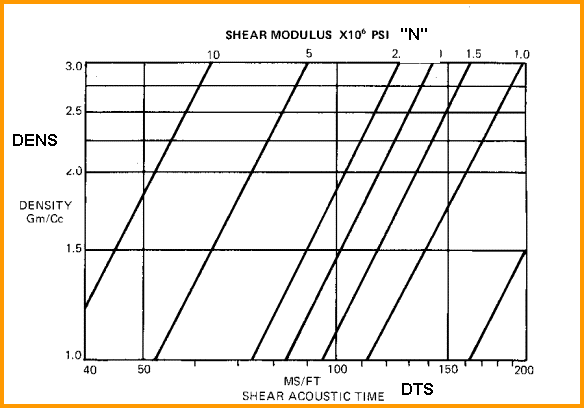Chart to calculate N from DENS and DTSPoisson's Ratio PR (aka v or nu) is the lateral strain divided by longitudinal strain.

When shear velocity or shear travel time is available:
For rock with porosity:
1: R = Vp / Vs
OR 2: R = DTS / DTC
3: PR = (0.5 * R^2 - 1) / (R^2 - 1)

For rock with no porosity:
4: Ro = DTSMA / DTCMA
5: PRo = (0.5 * Ro^2 - 1) / (Ro^2 - 1)

If the rock is anisotropic, PR can be calculated for the minimum and maximum stress directions by using DTSmin and DTSmax from a crossed dipole shear sonic log. PRmax comes from DTSmin and vice versa.

When shear travel time is not known, which is the case in the vast majority of older wells, a value for Poisson's ratio can be estimated. The usual estimate for Poisson's ratio in shaly sands is:
6: PR = 0.125 * Vsh + 0.27

This was developed in the US Gulf Coast and the parameters might need some adjustment in other areas.

A table of values for other rock types is shown later in this section.

If good conventional and shear seismic data are available, then Poisson's ratio can be derived continuously from seismic data. This is sometimes referred to as “seismic petrophysics”.

For quicklook analysis, use this chart for Poisson’s Ratio: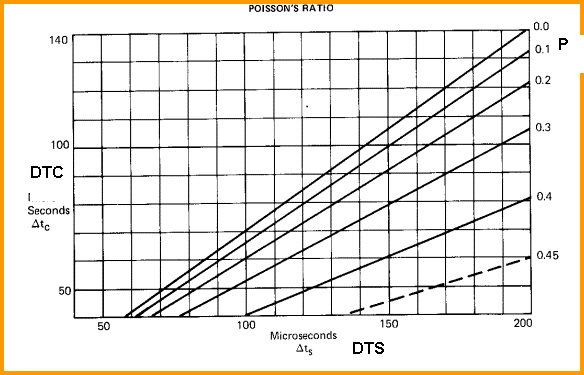Chart to calculate PR from DTC and DTS

A plot of Poisson's ratio versus compressional velocity, below, shows the effect of lithology and gas. Values for Poisson's ratio are also listed in Table 1 near the end of this Chapter.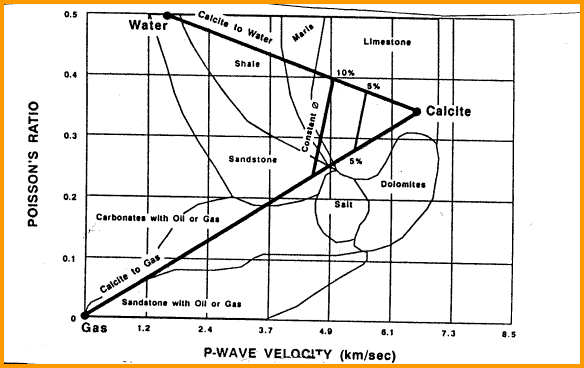Poisson’s ratio versus lithology

In the absence of good shear sonic data, Poison's Ratio can be estimated from the graph below, based on known or assumed lithology (courtesy Barree and Associates).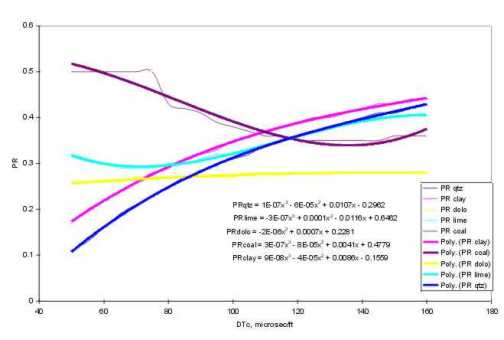Correlations of Poisson's Ratio versus DTC

The equations on this graph are:
7: PRshl = min(0.5,max(0,0.000 000 086 754 * DTC^3 - 0.000 044 154 * DTC^2 + 0.008 587 * DTC - 0.155))
8: PRqrtz = min(0.5,max(0,0.000 000 126 482 * DTC^3 - 0.000 058 769 * DTC^2 + 0.010 703 * DTC - 0 .296))
9: PRlime = min(0.5,max(0,-0.000 000 341 745 * DTC^3 + 0.000 117 836 * DTC^2 - 0.011 609 * DTC + 0.646))
10: PRdolo = min(0.5,max(0,-0.000 002 394 128 * DTC^2 + 0.000 708 300 * DTC + 0.2281355))
11: PRcoal = min(0.5,max(0.32,0.000 000 334 448 * DTC^3 - 0.000 083 251 * DTC^2 + 0.004 122 * DTC + 0.478))
12: PR = SUM(Vi * PRi)

Where:
DTC = sonic travel time (usec/ft)
Vi = volume of each component of the ock (fractional)
PRi = Poisson's Ratio of each component (fractional)

NOTE: Be sure to convert metric DTC values to Engliah units.

A high Poisson’s ratio indicates high stress level, which in turn indicates possible boundaries to a hydraulic fracture. Low Poisson’s ratio indicates weak zones which may not constrain the frac job, resulting in communication to undesired formations. Most shales constrain fractures but some may not do so. Two to three meters of rock with a Poisson's Ratio greater than 0.26 is the minimum needed to constrain a typical hydraulic fracture.

Gas zones, where the sonic compressional data has not been corrected for gas, will show abnormally low Poisson's ratio.

Poisson’s ratio is used to predict fracture pressure gradient in consolidated formations (Section 20.10).

 Typical Poisson's Ratio values Sandstone 0.18 - 0.22 deeper, highly compacted, cemented Sandstone 0.22 - 0.40 shallow, uncompacted, poorly cemented Siltstone 0.20 - 0.28 Shale 0.26 - 0.40 Limestone 0.310 Dolomite 0.283 Anhydrite 0.319 NOTE: Gas and Organic Carbon (TOC) lower these values.Bulk Modulus is the hydrostatic pressure divided by volumetric strain.
For rock with porosity:
1: Kb = KS5 * DENS *(1 / (DTC^2) - 4/3 * (1 / (DTS^2)))

For rock with no porosity:
2: DENSMA = (DENS - PHIt * DENSW) / (1 - PHIt)
3: DTCMA = (DTC - PHIt * DTW) / (1 - PHIt)
4: Km = KS5 * DENSMA * (1 / (DTCMA ^ 2) - 4/3 * (1 / (DTSMA^2)))

Where:
KS5 = 13400 for English units
KS5 = 1000 for Metric units

If the rock is anisotropic, both Kb and Km can be calculated for the minimum and maximum stress directions by using DTSmin and DTSmax from a crossed dipole shear sonic log.

Density is in gm/cc, travel time is in usec/ft, and Kb is in psi * 10^6 for English units. Density is in kg/m3, travel time is in usec/m, and Kb is in Giga-Pascals (10^9 Pa or GPa) for Metric units.

If you like quicklook charts, here is one for Kb:Chart for calculating Kb from P and NBulk Compressibility is the inverse of Bulk Modulus.

For rock with porosity:
1: Cb = 1 / Kb

For rock with no porosity:
2: Cm = 1 / Km

This term is called rock compressibility and abbreviated Cr in some literature.

If the rock is anisotropic, both Cb and Cm can be calculated for the minimum and maximum stress directions by using DTSmin and DTSmax from a crossed dipole shear sonic log.

N and Cb predict sanding (sand production) in unconsolidated formations. When log analysis shows sanding may be a problem, sand control methods (injection of plastic or resin or gravel packing) can be initiated. Sanding is not a problem when N > 0.6*10^6 psi. in oil or gas zones. High water cuts increase the likelihood of sanding. This threshold corresponds to Cb of 0.75*10^-6 psi^-1. N/Cb > 0.8*10^12 psi^2 is a more sensitive cutoff than either N or Cb cutoffs. High N/Cb ratios indicate low chance for sanding. A good cement job is also needed to reduce sanding.Biot's Constant is the ratio of the volume change of the fluid filled porosity to the volume change of the rock when the fluid is free to move out of the rock (ie. the hydraulic pressure remains unchanged)..

For rock with porosity:
1: ALPHA = 1 - Kb / Km
OR 2: ALPHA = 1 - Cm / Cb

For rock with no porosity, Kb = Km so ALPHA = 0.

If shear travel time is unavailable, this empirical relation may be useful:
3: ALPHA = 1 - (1 - PHID) ^ KS8

where KS8 has the range 2 to 3, with KS8 = 3 most often used.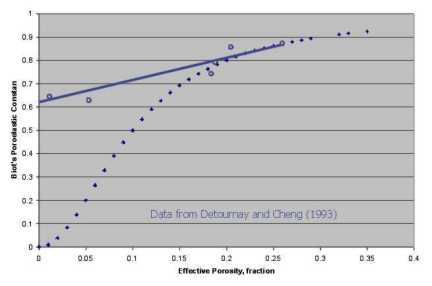Biot's Constant versus porosity

In the absence of good shear sonic data, Biot's Constant can be estimated from the graph above, based on known or assumed lithology (courtesy Barree and Associates). This graph suggests KS8 in the previous equation is greater than 2.0. The empirical straight line fit to the data is:
4: ALPHA = 0.62 + 0.935 * PHIeYoung's Modulus is applied uni-axial stress divided by normal strain.

For rock with porosity:
1: Y = 2 * N * (1 + PR)

For rock with no porosity:
2: Yo = 2 * No * (1 + PRo)

If the rock is anisotropic, Y can be calculated for the minimum and maximum stress directions by using DTSmin and DTSmax from a crossed dipole shear sonic log when calculating N and P.

Young's modulus calculated from log data is often called the dynamic Young's modulus, Ydyn.

Young’s modulus is used in the fracture width (aperture) calculation in fracture design software.

Here is the quicklook chart for Young’s modulus: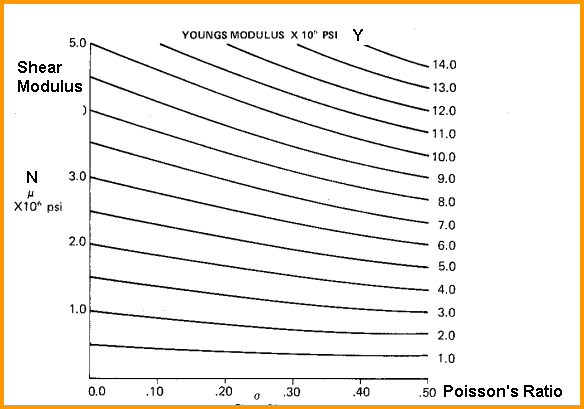Chart to calculate Y from PR and N

In the absence of good shear sonic data, Young's Modulus can be estimated from the graph below, based on known or assumed lithology (courtesy Barree and Associates). The ordinate on this graph is Young's Modulus divided by density (gm/cc), so multiply the Y axis value by density to obtain Y.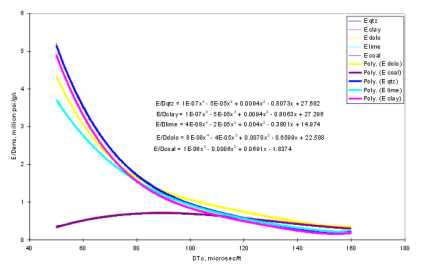Young's Modulus versus DTC for various lithologies

The equations on the above graph are:
3: Yshl = 6.894 * DENS * (0.000 000 100 214 * DTC^4 - 0.000 050 013 * DTC^3 + 0.009 417 * DTC^2
- 0.806 315 * DTC + 27.30)
4: Yqrtz = 6.894 * DENS * (0.000 000 099 297 * DTC^4 - 0.000 049 604 * DTC^3 + 0.009 3678 * DTC^2
- 0.807 280 * DTC + 27.68)
5: Ylime = 6.894 * DENS * (0.000 000 037 682 * DTC^4 - 0.000 019 762 * DTC^3 + 0.003 996 * DTC^2
- 0.380 084 * DTC + 14.97)
6: Ydolo = 6.894 * DENS * (0.000 000 084 048 * DTC^4 - 0.000 041 6941 * DTC^3 + 0.007 775 * DTC^2
- 0.659 893 * DTC + 22.59)
7: Ycoal = 6.894 * DENS * (0.000 001 498 * DTC^3 - 0.000 588 141 * DTC^2 + 0.069 142 * DTC - 1.84)
8: Ydyn = SUM(Vi * Yi)

Where:
DTC = sonic travel time (usec/ft)
DENS = density (g/cc)
Vi = volume of each component of the rock (fractional)
Yi = Young's Modulus of each component (GPa)
Ydyn = dynamic Young's Modulus of rock (GPa)

NOTE: Be sure to convert metric DTC and DENS values to English units. Results are in GPa. Divide by 6.894 to get psi * 10^6.Brittleness Indicators
Lame's Constant L, (aka λ (lambda)) is a measure of a rocks brittleness, which is a function of both Young's Modulus and Poisson's Ratio:
1: L = Y * PR / ((1 + PR) * (1 - 2 * PR))
OR 1A: L = DENS * (Vp^2 - 2 * Vs ^ 2)

Where:
L = Lame's Constant  (psi * 10^6 or KPa)
Y = Young's Modulus (psi * 10^6)
PR = Poisson's Ratio (unitless)

Young's Modulus vs Poison's Ratio: Brittleness increases toward top left, density increases toward top right, porosity plus organic content and depth decrease toward bottom left. PR values less than 0.17 indicate gas or organic content or both. (image courtesy Canadian Discovery Ltd)

Mullin proposed a practical model that is widely used as a brittleness indicator (SPE 115258, 2007):
2: Y1 = ((Yst - 1) / (8 - 1) * 100)
3: PR1 = ((PR - 0.40) / (0.15 - 0.40)) * 100
4: BImullin = (Y1 + PR1) / 2

Where:
Yst = static Young's Modulus (psi * 10^6)
PR = Poisson's Ratio (unitless)
Y1 = Young's Modulus for brittleness calculation
PR1 = Poisson's Ratio for brittleness calculation
BImullin = Mullen's Brittleness Coefficient

Note that Young's Modulus in the Mullin equation is the static value (see next Section) and must be in
psi * 10^6. Divide Y in GPa by 6.894 to get Y in psi * 10^6. The no porosity case can be generated by replacing Y and PR with their no porosity values.

Glorioso offers a direct brittleness indicator based solely on the lithologic composition of the rock (SPE 153004, 2012).
5: BIlith = (Vqrtz + Vlime + Vdolo + Vanhy) / (Vqrtz + Vlime + Vdolo + Vanhy + Vsh + Vker)

Where:
BIlith = brittleness index from lithology
Vxxx = mineral volume fraction relative to total mineral volume. (fractional)

This paper was specifically written for shale gas cases, which have naturally low porosity,  and this index may not be useful in more porous rocks unless a porosity term is added to the denominator.Modulus of Compressibility
Fluid bulk modulus:
1: Kf = 1/Cf = Sw / Cwtr + (1 - Sw) / Chyd

Pore bulk modulus:
2: Kp = ALPHA^2 / ((ALPHA - PHIt) / PHIt / Kf)

For rock with porosity:
3: Kc = Kp + Kb + 4/3 * N.

For rock with no porosity, Kp = 0 and Kb = Km, and N = No, so:
4: Kc  = Km + 4/3 * No

 RECOMMENDED PARAMETERS: Water Salinity Cf psi-1 Kf psi Cf GPa-1 Kf GPa 5000 0.0000040 250000 0.580 1.723 35000 0.0000039 270270 0.537 1.862 200000 0.0000027 344828 0.420 2.376 Oil Depth 2000 ft 610 m 0.0000085 117647 1.233 0.811 4000 ft 1220 m 0.0000095 105263 1.378 0.725 8000 ft 2440 m 0.0000116 86207 1.683 0.594 12000 ft 3660 m 0.0000135 74074 1.959 0.510 Gas Depth 2000 ft 610 m 0.001250 800 181.422 0.006 4000 ft 1220 m 0.000510 1961 74.020 0.014 8000 ft 2440 m 0.000180 5556 26.124 0.038 12000 ft 3660 m 0.000100 10000 14.513 0.069

The above calculations assume that fluid compressibilities are known from lab measurements of produced fluids. In recently drilled wells, this information is not always available. It would therefore be useful to predict fluid compressibility or fluid bulk modulus and use this result to predict the fluid type in the reservoir. A method using pore bulk modulus is more convenient, and is based on some empirical evidence for sandstones.

By setting Kb = Km - 0.9 * N in equation 3, and solving for Kp:
5: Kp = Kc - Km + 0.9 * N - 4/3 * N

Interpretation of Kp is based on the following:
6: IF Kp <= 0.15 THEN Zone is gas bearing (Kp in GPa):
7: IF 0.15 < Kp < 0.35 THEN Zone is oil bearing
8: IF Kp >= 0.35 THEN Zone is water bearing

Kp is sometimes shown as Kf in the literature so be careful.Quicklook Bulk and Shear Modulus
Murphy (1991) provided equations for sandstone rocks (PHIe < 0.35) that predict Kb and N from porosity:
1: Kb = 38.18 * (1 - 3.39 * PHIe + 1.95 * PHIe^2)
2: N   = 42.65 * (1 - 3.48 * PHIe + 2.19 * PHIe^2)

These equations are for the water filled case and cannot be used as fluid identification, but they may have other uses.Calibrating Dynamic to Static Constants
The mechanical properties of rocks derived from log data, or from high frequency sonic measurements in the lab are called dynamic constants. Those derived in the laboratory from stress strain tests or destructive tests are called static constants. In my opinion, lab derived dynamic shear data should be corrected for high frequency effects, as well. This is seldom done, so it is difficult to compare dynamic log data to dynamic lab data.

Unfortunately, the difference between dynamic (well log) values and static (lab) values on cores can be quite large, leading some people to dismiss the log data as wrong or useless. What makes this worse is that fracture design software has been calibrated to static (lab derived) values, so dynamic data has to be transformed to equivalent static numbers.

Static values differ from dynamic values because wave propagation is a phenomenon of small strain with a large strain rate: rocks appear stiffer in response to an elastic wave, compared to a rock mechanics laboratory (triaxial) test, where larger strains are applied at lower strain rate. The weaker the rock, the larger the difference between elastic properties derived from acoustic measurements (dynamic) and those derived from triaxial measurements (static). This accounts for the marked difference between dynamic and static Young’s moduli. However, the difference between dynamic and static Poisson’s ratio is very small, and is generally not considered.

There are further considerations. Since the tiny core plugs used for lab work have been de-stressed and re-stressed a number of times, there is some doubt that this cycle is truly reversible, so lab measurements may not represent in-situ conditions. The difference between static and dynamic values are larger for higher porosity, which suggests that some grain bonds are easily broken by coring and subsequent testing. It might be a wise move to calibrate fracture design software to dynamic data, since this data is more readily available, and may actually have fewer inherent measurement problems.

Further, the effects of reservoir anisotropy cannot be simulated in the lab, so there is no reason to expect lab data to match in-situ log results. A possible solution to this dilemma is described in later Sections.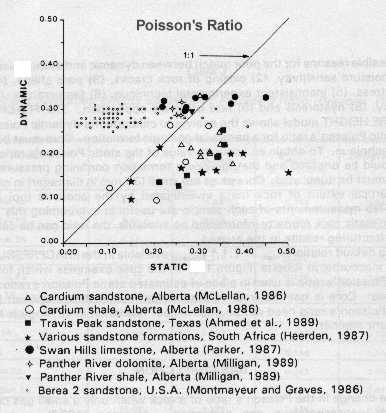Errors in Poisson's ratio strongly affect calculation of in-situ closure stress.

Doug Boyd (1991) presented a summary of published Poisson's Ratio data, shown at the right.

As you can see, there is no direct relation between dynamic and static Poisson' ratio. Additional reasons for the mismatch might include dry rock measurements (as opposed to restored state), moisture variations in shaly samples, closing of fractures, pore shape changes, anisotropy (especially if core plugs are used instead of whole core), inconsistent (and often unknown) experimental methods, and experimental measurement error. Results of such a comparison made today might be less erratic if anisotropic effects were reduced by use of crossed dipole shear sonic data and appropriate core handling procedures.

Calibration of local data seems possible, but there is no universal correction factor. When field measured closure stress is available from mini-fracs, a calibration of Poisson's Ratio is fairly easy in a homogenous reservoir, but probably impractical in many cases.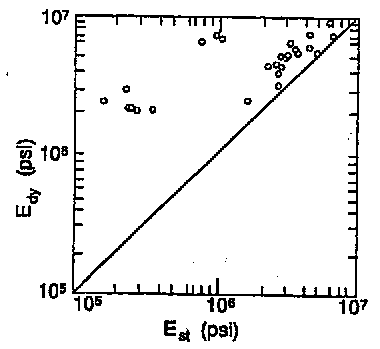Young’s modulus is also affected by differences between static and dynamic values. A transform published by Morales and Marcinew in 1993 is shown in the graph on the left and formulated as:

1: Yst = 10^(A + B * log(Ypsi))

Where:
Yst = static Young’s modulus (psi)
Ypsi = dynamic Young’s modulus (psi)

A and B are constants that depend on porosity as shown below:

 Porosity A B 0.00 - 0.10 n/a 0.10 - 0.15 2.137 0.6612 0.15 - 0.25 1.829 0.6920 > 0.25 -0.4575 0.9402

This transform was based on high frequency dynamic lab data compared to static lab data. Low frequency log data was not used so this widely used transform may have no validity for log derived results.

The same paper quoted other data sets and compared their data to a transform by Eissa. Note that these transforms invoke the rock density to normalize the data. The equations are:

Average of all data
2: Yst = 10^(0.05 + 0.77 * log(DENS * Ydyn))

Low porosity 10 - 15%
3: Yst = 10^(0.02 + 0.77 * log(DENS * Ydyn))

Medium porosity 15 - 25%
4: Yst = 10^(-0.11 + 0.77 * log(DENS * Ydyn))

High porosity > 25%
5: Yst = 10^(-0.72 + 0.77 * log(DENS * Ydyn))

NOTE: DENS is in g/cc and Ydyn is in GPa.Static to dynamic transforms for Young's Modulus

Lacy proposed a correlation to obtain static Young's Modulus, Yst, from Ydyn. First convert Ydyn to English units:
6: Ypsi = Ydyn / 6.894
7: Yst_shl = 6.894 * (0.0420 * Ypsi^2+0.2330 * Ypsi)
8: Yst_qrtz = 6.894 * (0.0293 * Ypsi^2 + 0.4533 * Ypsi)
9  Yst_carb = 6.894 * (0.0180 *Ypsi^2 + 0.4220 * Ypsi)
10: Yst_coal = Ycoal
11: Yst_lacy = SUM(Vi * Ysti)

NOTE: Results are in GPa. Divide by 6.894 to get psi * 10^6.

Barba's correlation for Yst is:
12: Y99 = min(0.9,max(0.5,(Vsh+Vqrtz)*(-0.0003 * Ypsi^4 + 0.0052 * Ypsi^3 - 0.0203 * Ypsi^2 + 0.0312 * Ypsi
+ 0.4765) + ((Vlime + Vdolo) * (Ypsi * 0.85 - 0.424)) / (Vsh + Vrock)))
13: Yst_barba = Y99 * Ydyn

NOTE: Results are in GPa. Divide by 6.894 to get psi * 10^6.ROCK STRENGTH
The static Young’s modulus is used to get the static uniaxial compressive strength (STRc).
1: STRc = (3 + 4.1 * Yst) * 145

Where,
Yst = static Young’s modulus  (psi)
STRc = uniaxial compressive strength (psi)

The tensile strength (STRt) is obtained from the UCS by
2: STRt = KST1 * STRc

Where:
KST1 = 0.08 to 0.10
STRt = tensile strength (psi)Examples of Mechanical Properties Logs
The above equations can be computed continuously and presented as logs. The format and curve complement vary widely between service companies and age of log. Some logs have Metric depths but the moduli are in English units. Some are vice versa. Here are some examples.Dynamic elastic properties calculated from density and sonic log data, showing close match to dynamic data from lab measurements (coloured dots). Note synthetic sonic and density plotted next to measured log curves (Tracks 2 and 3), showing reasonably small differences due to minor borehole effects. Synthetic curves can repair worse logs or even replace missing curves.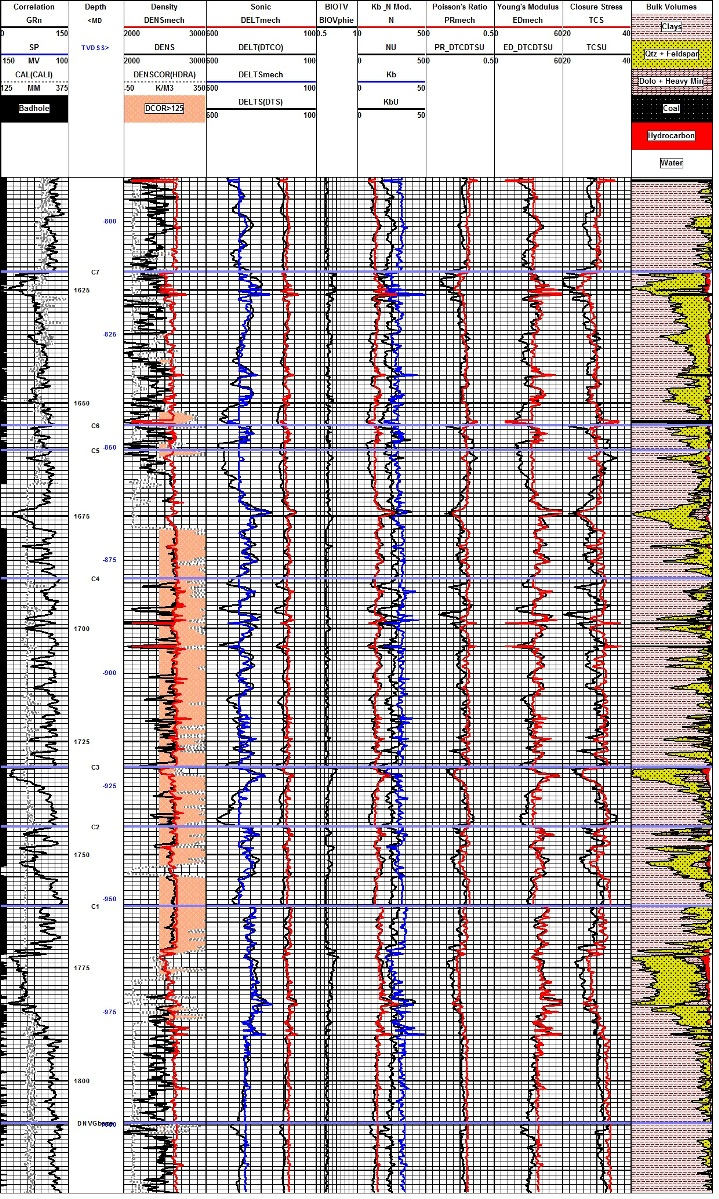Example of log reconstruction in a shaly sand sequence (Dunvegan). The 3 tracks on the left show the measured gamma ray, caliper, density, and compressional sonic. Original density and sonic are shown in black, modeled logs are in colour. Shear sonic is the model result as none was recorded in this well. Computed elastic properties are shown in the right hand tracks. Results from the original unedited curves are shown in black, those after log editing are in colour. Note that the small differences in the modeled logs compared to the original curves propagate into larger differences in the results, especially Poisson's Ratio (PR), Young's Modulus (ED), and total closure stress (TCS).

Page Views ---- Since 01 Jan 2015
Copyright 2023 by Accessible Petrophysics Ltd.
CPH Logo, "CPH", "CPH Gold Member", "CPH Platinum Member", "Crain's Rules", "Meta/Log", "Computer-Ready-Math", "Petro/Fusion Scripts" are Trademarks of the Author